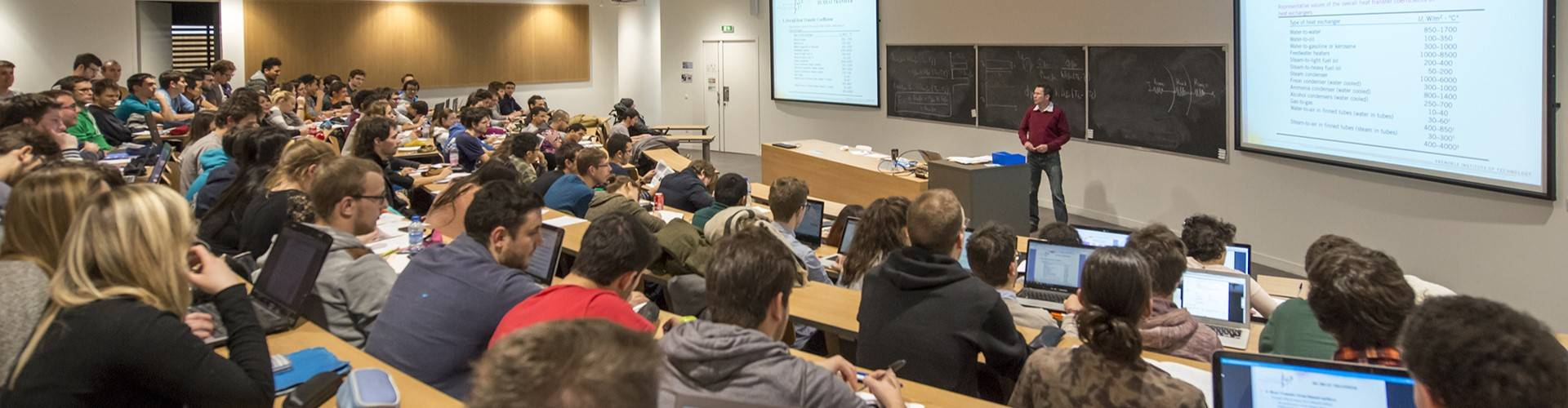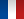# Applied Fluid Mechanics for the Environment - 4EUS3MFE

• #### Number of hours

• Lectures 25.0
• Projects -
• Tutorials 25.0
• Internship -
• Laboratory works 10.0
• Written tests 0

ECTS 5.0

## Goal(s)

The course is composed in three parts :
- turbulence: physical and modelling aspects
- diffusion and dispersion in the Environment
- environmental fluid mechanics

Responsible(s)

Olivier METAIS

## Content(s)

Turbulence: physical and modelling aspects(12 h of lecture and 4h of supervised work)
Content:
o Turbulent flows characteristics
o Statistical approach : averaging, Reynolds equations ; turbulent eddy-viscosity and diffusivity ; turbulent boundary layer ; energy mechanisms: production and dissipation
o Statistical tools and theories : correlations ; Fourier space ; kinetic energy and dissipation spectra ; turbulent scales ; Kolmogorov theory
o Turbulent shear flows : example of the plane jet
o The different approaches for turbulence modelling and simulation: statistical modelling, direct numerical simulation, large eddy simulation
o Statistical modelling: concept of order ; zero, one and two-equations models ;
k-epsilon model; second order model
o Large-eddy simulation : methodology ; Smagorinsky model

Diffusion and dispersion in the Environment (6 h of lecture et 2h of supervised work)
Content :
o Diffusion concept and Fick’s law : diffusion equation ; molecular and turbulent diffusion coefficients
o Unidirectional diffusion problem : concentrated injection ; extended injection ; presence of walls ; diffusion in a current
o Multidirectional diffusion problems : concept of plume
o Dispersion in shear flows : longitudinal dispersion coefficient
o Application to turbulent flows in rivers : characteristic turbulent velocity and velocity profile ; mixing distances in rivers ; flow rate determination
o Diffusion in fully-developed turbulence: diffusion of a cloud of tracers ; particle dispersion ; dispersion of particles ; Taylor’s theorem ; Richardson law .

Environmental fluid mechanics (12h of lecture et 12h of supervised work)
Content :

• Dimensional analysis and Vashy-Buckingham theorem
• Laminar boundary layer : Blasius equation and resolution methods ; boundary layer with adverse pressure gradient and separation
• Energy equation ; Equation de l’énergie ; Compressible flow statics and concept of atmospheric stability
• Rotating flows ; centrifugal and Coriolis forces ; Ekman layer
• Vorticity dynamics
12h of practical activities

Prerequisites

Basis in Fluid mechanics

Test

Session normale / First session
Evaluation non rattrapable (EN) / EN assessment
Evaluation rattrapable (ER) / ER assessment : épreuve écrite de 4h / 4h written exam

Session de rattrapage / Second session
La note remplace la note de ER. Le EN n'est pas rattrapable. / Written or oral exam will replace the first one (ER). No retake for EN.

EN*1/3 + ER*2/3

Calendar

The course exists in the following branches:

see the course schedule for 2023-2024

Course language(s):